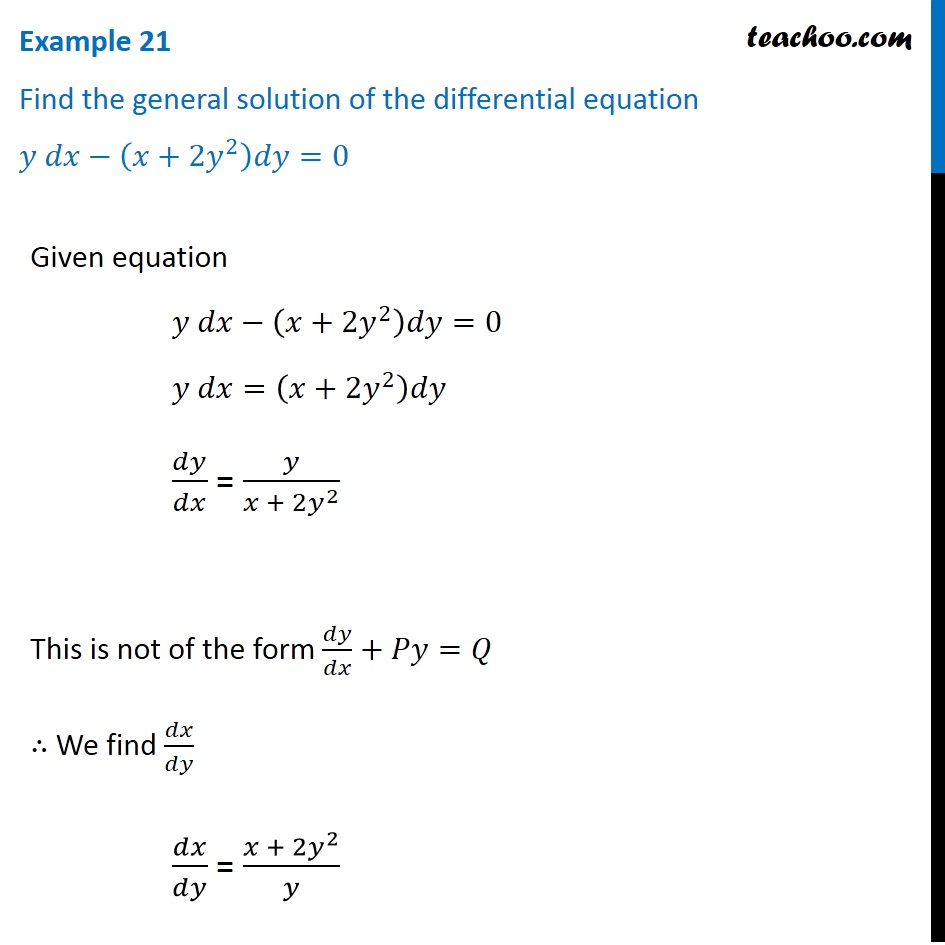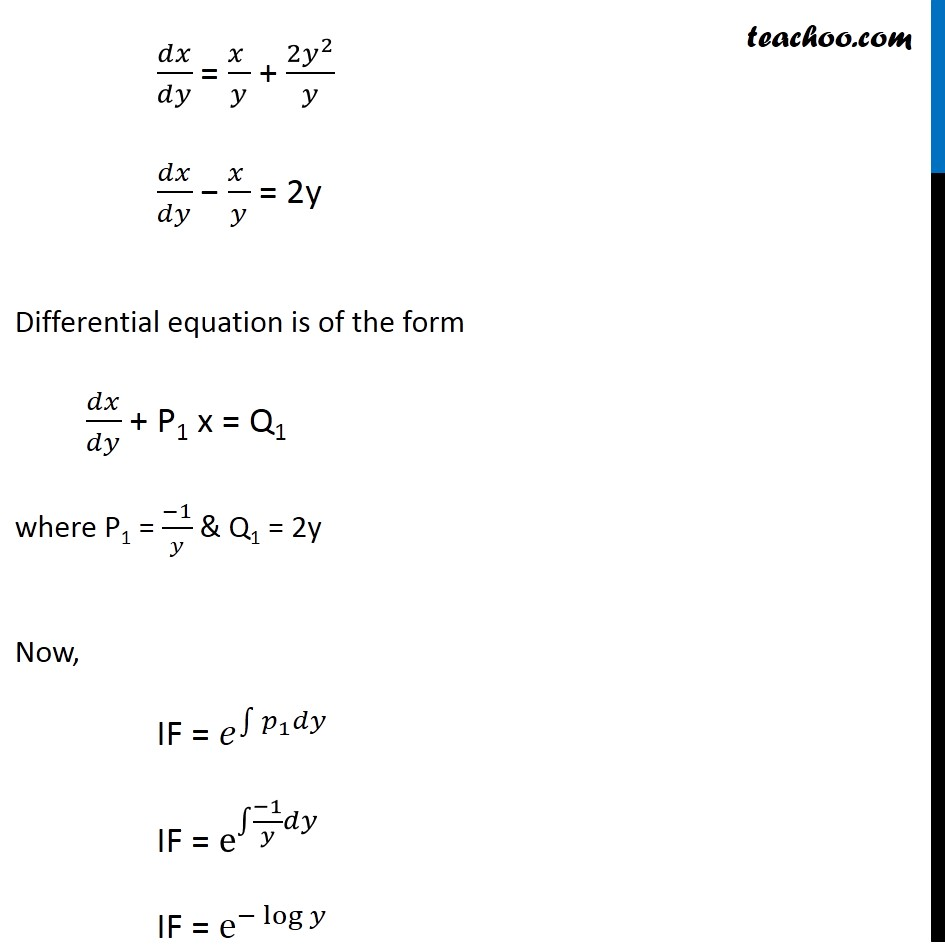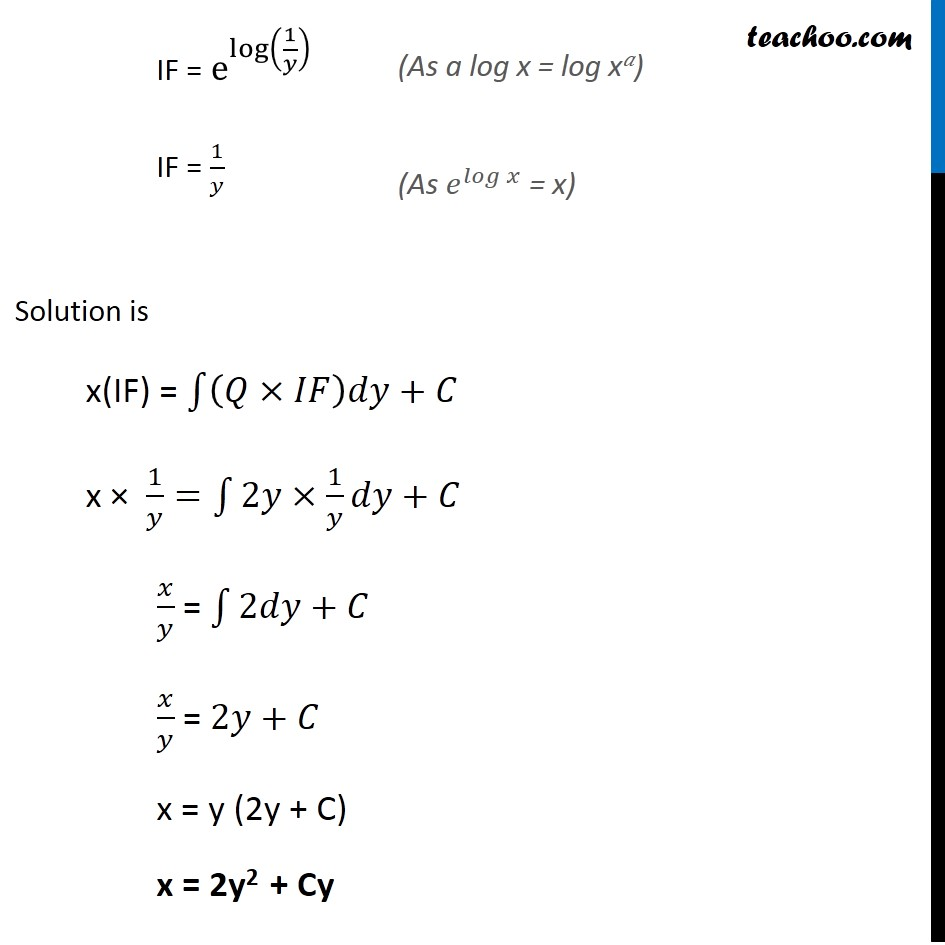Examples

Chapter 9 Class 12 Differential Equations
Serial order wiseLearn in your speed, with individual attention - Teachoo Maths 1-on-1 Class

### Transcript

Example 16 Find the general solution of the differential equation 𝑦 𝑑𝑥−(𝑥+2𝑦^2 )𝑑𝑦=0 Given equation 𝑦 𝑑𝑥−(𝑥+2𝑦^2 )𝑑𝑦=0 𝑦 𝑑𝑥=(𝑥+2𝑦^2 )𝑑𝑦 𝑑𝑦/𝑑𝑥 = 𝑦/(𝑥 + 2𝑦^2 ) This is not of the form 𝑑𝑦/𝑑𝑥+𝑃𝑦=𝑄 ∴ We find 𝑑𝑥/𝑑𝑦 𝑑𝑥/𝑑𝑦 = (𝑥 + 2𝑦^2)/𝑦 𝑑𝑥/𝑑𝑦 = (𝑥 )/𝑦 + (2𝑦^2)/𝑦 𝑑𝑥/𝑑𝑦 − (𝑥 )/𝑦 = 2y Differential equation is of the form 𝑑𝑥/𝑑𝑦 + P1 x = Q1 where P1 = (−1)/𝑦 & Q1 = 2y Now, IF = 𝑒^∫1▒〖𝑝_1 𝑑𝑦〗 IF = e^∫1▒〖(−1)/𝑦 𝑑𝑦" " 〗 IF = e^(−log⁡𝑦 ) IF = e^log⁡(1/𝑦) IF = 1/𝑦 IF = e^log⁡(1/𝑦) IF = 1/𝑦 Solution is x(IF) = ∫1▒〖(𝑄×𝐼𝐹)𝑑𝑦+𝐶 〗 x × 1/𝑦=∫1▒〖2𝑦×1/𝑦〗 𝑑𝑦+𝐶 𝑥/𝑦 = ∫1▒〖2𝑑𝑦+𝐶〗 𝑥/𝑦 = 2𝑦+𝐶 x = y (2y + C) x = 2y2 + Cy (As a log x = log x𝑎) (As 𝑒^𝑙𝑜𝑔⁡𝑥 = x)